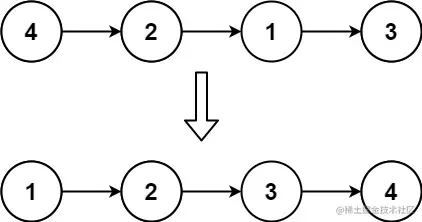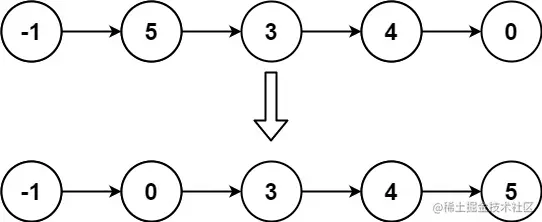# leetcode 147. Insertion Sort List（python）

2022-02-01 10:39:44

「 This is my participation 11 The fourth of the yuegengwen challenge 26 God , Check out the activity details ：2021 One last more challenge

### describe

Given the head of a singly linked list, sort the list using insertion sort, and return the sorted list's head.

The steps of the insertion sort algorithm:

• Insertion sort iterates, consuming one input element each repetition and growing a sorted output list.
• At each iteration, insertion sort removes one element from the input data, finds the location it belongs within the sorted list and inserts it there.
• It repeats until no input elements remain.

The following is a graphical example of the insertion sort algorithm. The partially sorted list (black) initially contains only the first element in the list. One element (red) is removed from the input data and inserted in-place into the sorted list with each iteration.

Example 1:``````Input: head = [4,2,1,3]
Output: [1,2,3,4]
Copy code ``````

Example 2:``````Input: head = [-1,5,3,4,0]
Output: [-1,0,3,4,5]
Copy code ``````

Note:

``````The number of nodes in the list is in the range [1, 5000].
-5000 <= Node.val <= 5000
Copy code ``````

### analysis

According to the meaning , Given a one-way linked list head , Use insert sort to sort the linked list , And return the sorted list head .

Insert the steps of sorting algorithm ：

• Insert sort to iterate , Consume one input element at a time and generate a sorted output list
• In each iteration , Insert sort removes an element from the input data , Find its location in the sort list and insert it there
• Repeat until no input elements are left

The insertion order given by the title is rather obscure , In fact, the content of the investigation is very basic , Is the search and insertion of linked list nodes , Every time we traverse a new node , Find the place where it should be inserted in the front linked list that has been ordered , Insert the node . The key is to maintain the different pointers that need to be used , Just don't get dizzy .

``````class ListNode(object):
def __init__(self, val=0, next=None):
self.val = val
self.next = next
class Solution(object):
"""
:rtype: ListNode
"""
L = ListNode(val=-10000)
L.next = curr = head
while curr.next:
pre = L
if curr.next.val>=curr.val:
curr = curr.next
continue
while pre.next.val<curr.next.val:
pre = pre.next
tmp = curr.next
curr.next = tmp.next
tmp.next = pre.next
pre.next = tmp
return L.next

Copy code ``````

### Running results

``````Runtime: 172 ms, faster than 81.63% of Python online submissions for Insertion Sort List.
Memory Usage: 17.2 MB, less than 15.65% of Python online submissions for Insertion Sort List.
Copy code ``````